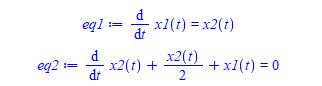## 5696 Reputation

8 years, 297 days

## MaplePrimes Activity

### These are answers submitted by nm

dsolve gives answer with integrals it could not evaluate. Try numerical

```restart;
sys := diff(x1(t), t) = 3*x1(t) - 2/1000*x1(t)*x2(t), diff(x2(t), t) = 6/10000*x1(t)*x2(t) - 1/2*x2(t);
ics := x1(0) = 1000, x2(0) = 500;
sol:=dsolve([sys,ics],numeric,{x1(t),x2(t)},output=listprocedure);
plot(rhs(sol)(t),t=0..4,labels=["t","x1"]);
plot(rhs(sol)(t),t=0..4,labels=["t","x2"]);

```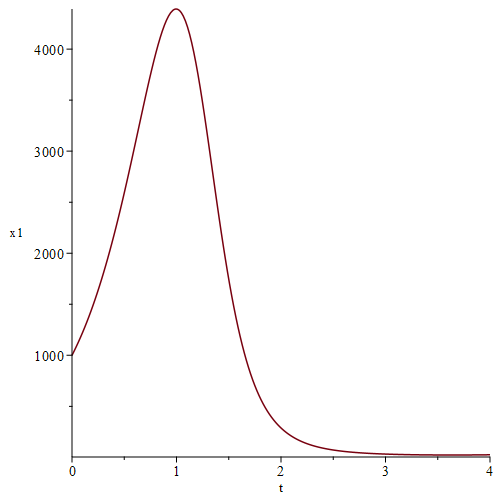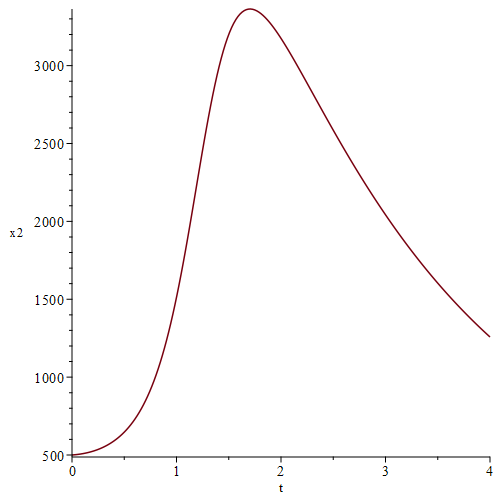one possibility could be

```restart;
G[i,k]:=u[i] - u[k]-(u[i]-u[k])^2;
```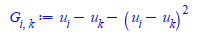```for i from 1 to 3 do
for j from 1 to 3 do
for k from 1 to 3 do
G[i,k]:=u[i] - u[k]-(u[i]-u[k])^2;
od;
od;
od;

G[2,1];
G[3,2];
```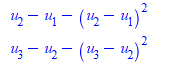its latex

```latex(G[2,3])

G_{2,3}```

double underscore could have worked  but do not know how to make it work with 2 indices (i,j).

For example , for the RHS you could do

`u__i- u__k-(u__j-u__k)^2`

But this would not work for G with double index.

You should really post full code you used, including the plot command used.

It works for me on Maple 2021. no cut off.

```restart;
h := x -> 1.23 + x*1*0.0001 + 0.12*log(50000*x) + abs((-1)*0.03*log(x/0.001));
plot(h(x),x=0..100)
```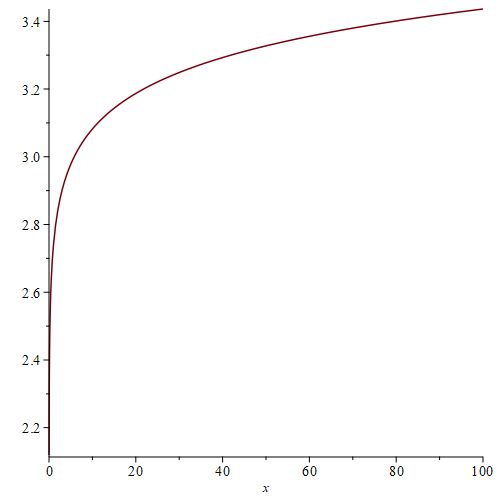One possible way is to use the solve command. Also the PDEtools Solve command can do it.

```restart;
K1:=1;
K2:=2;
N:=3;
eq1:=K1= N*(R9 + R6) + R9 / (R9 + R6)*N^2 + (2*R6 + 2*R9)*N + R9;
eq2:=K2= N*(R6 + R9) / (R6 + R9)*N^2 + (2*R6 + 2*R9)*N + R9;
PDETools:-Solve([eq1,eq2],[R9,R6]);

evalf(%)```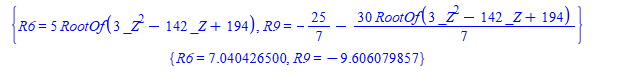```restart;
interface(version);

fname:="G:/data/tmp/ftest.mpl":
f:=proc(n)
local i,k;
for i to n do f(i):=parse(cat("", seq(k mod 10, k=1..10*i))) od;
f(n);
end:

f(80):
length(%);  # 800, ok
save f, fname;
f:='f':
#restart;
f(80):
length(%);  # 100  ???
```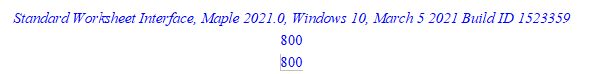one way might be to use solve or PDEtools:-Solve

```restart;
eq:=5*x^3-x^2+x-1=0;
PDEtools:-Solve(eq,x)
```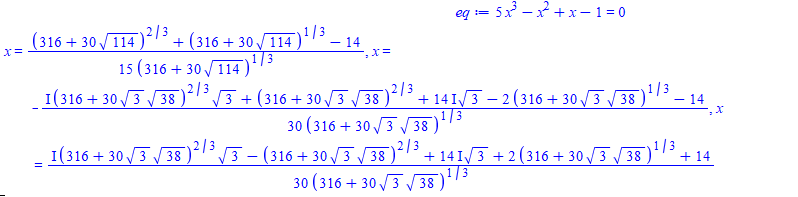second order ODE can be written as two first order ode's. So convert your second order ODE by introducting two state variables, x1(t) and x2(t). One for "position" and second for "speed".

Since you did not give an example of your second order ode's and its IC, I made one up.

```restart;
ode := diff(x(t),t\$2) +1/2*diff(x(t),t)+ x(t) = 0;
eq1:=diff(x1(t),t)=x2(t);
eq2:=subs([diff(x(t),t)=x2(t),x(t)=x1(t)],ode);
DEtools:-DEplot([eq1,eq2],[x1(t),x2(t)],t=0..35,[[x1(0)=1,x2(0)=1]],x1=-2..2,x2=-2..2,
numpoints=200, linecolor=black, axes=boxed);
```